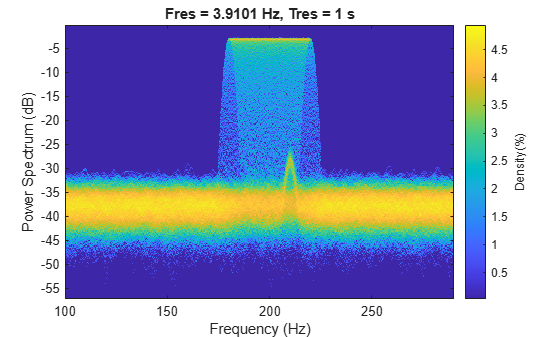# 时频分析实践介绍

### 使用时频分析识别 DTMF 信号中的数字```[tones, Fs] = helperDTMFToneGenerator(); p = audioplayer(tones,Fs,16); play(p)```

```N = numel(tones); t = (0:N-1)/Fs; subplot(2,1,1) plot(1e3*t,tones) xlabel('Time (ms)') ylabel('Amplitude') title('DTMF Signal') subplot(2,1,2) pspectrum(tones,Fs,'Leakage',1,'FrequencyLimits',[650, 1500])```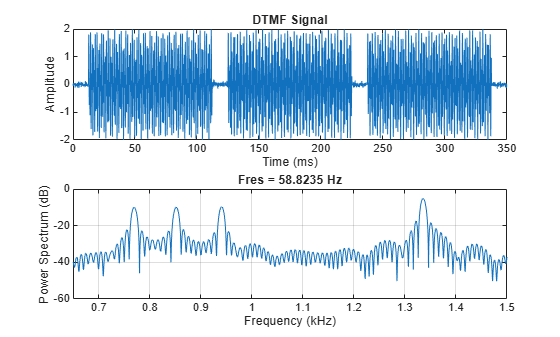```env = envelope(tones,80,'rms'); pulsewidth(env,Fs)```
```ans = 3×1 0.1041 0.1042 0.1047 ```
`title('Pulse Width of RMS Envelope')`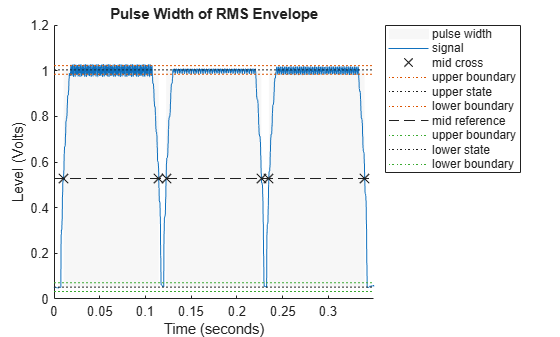```f = [meanfreq(tones,Fs,[700 800]), ... meanfreq(tones,Fs,[800 900]), ... meanfreq(tones,Fs,[900 1000]), ... meanfreq(tones,Fs,[1300 1400])]; round(f)```
```ans = 1×4 770 852 941 1336 ```

```pspectrum(tones,Fs,'spectrogram','Leakage',1,'OverlapPercent',0, ... 'MinThreshold',-10,'FrequencyLimits',[650, 1500]);```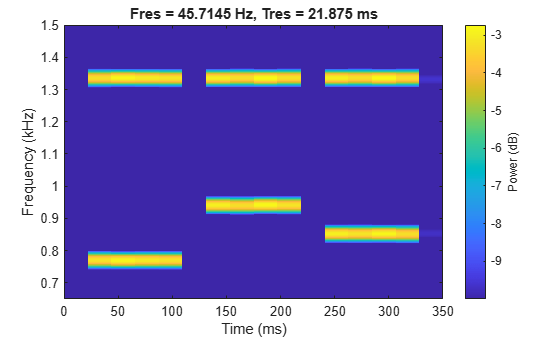### 权衡时间和频率分辨率以获得最佳的信号表示

`pspectrum` 函数将一个信号划分为若干个段。较长的段提供更好的频率分辨率；较短的段提供更好的时间分辨率。可以使用 `'FrequencyResolution'``'TimeResolution'` 参数控制段的长度。当未指定频率分辨率或时间分辨率值时，`pspectrum` 会尝试根据输入信号长度在时间分辨率和频率分辨率之间找到良好的平衡。

```load whaleTrill p = audioplayer(whaleTrill,Fs,16); play(p)```

```t = (0:length(whaleTrill)-1)/Fs; figure ax1 = subplot(3,1,1); plot(t,whaleTrill) ax2 = subplot(3,1,2); pspectrum(whaleTrill,Fs,'spectrogram','OverlapPercent',0, ... 'Leakage',1,'MinThreshold',-60) colorbar(ax2,'off') ax3 = subplot(3,1,3); pspectrum(whaleTrill,Fs,'spectrogram','OverlapPercent',0, ... 'Leakage',1,'MinThreshold',-60,'TimeResolution', 10e-3) colorbar(ax3,'off') linkaxes([ax1,ax2,ax3],'x')```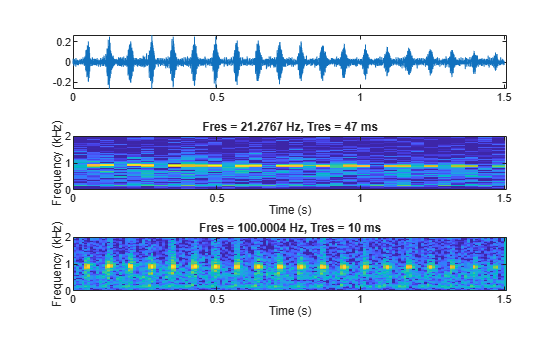`pspectrum` 选择的 47 毫秒时间分辨率不够小，不足以定位频谱图中的所有颤音脉冲。另一方面，10 毫秒的时间分辨率足以在时间上定位每个颤音脉冲。如果我们放大几个脉冲，这会变得更加清晰：

`xlim([0.3 0.55])`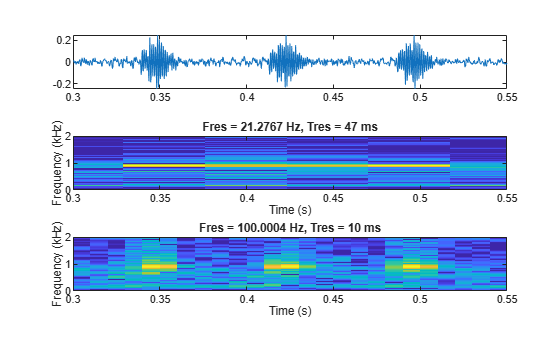```load batsignal Fs = 1/DT; figure pspectrum(batsignal,Fs,'spectrogram')```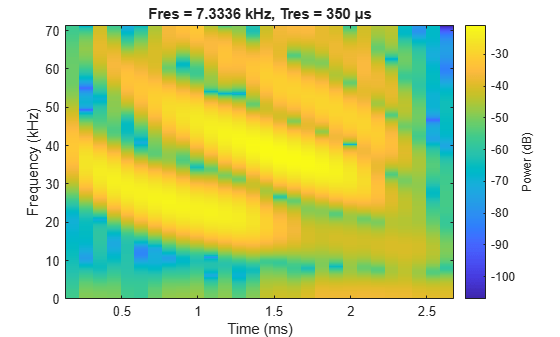`pspectrum(batsignal,Fs,'spectrogram','FrequencyResolution',3e3)`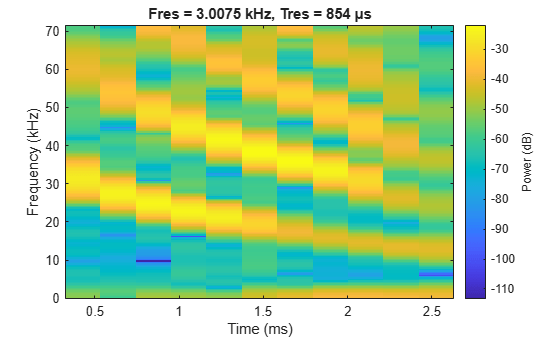```pspectrum(batsignal,Fs,'spectrogram','FrequencyResolution',3e3, ... 'OverlapPercent',99,'MinTHreshold',-60)```### 时频重排

`pspectrum` 函数能够在时间和频率上估计每个频谱估计值的能量中心。如果您将每个估计值的能量重新赋予最接近新时间和频率中心的 bin，您可以校正该窗的一些泄漏。您可以通过使用 `'Reassign'` 参数来完成此操作。将此参数设置为 `true` 会计算重排后的信号频谱图。

```pspectrum(batsignal,Fs,'spectrogram','FrequencyResolution',3e3, ... 'OverlapPercent',99,'MinTHreshold',-60,'Reassign',true)```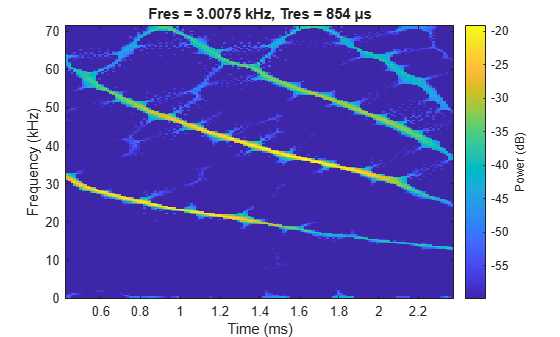### 重新构造时频脊线

```load splat p = audioplayer(y,Fs,16); play(p) pspectrum(y,Fs,'spectrogram')```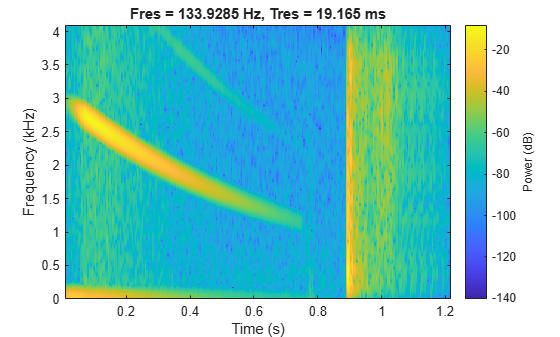```rng('default') t = (0:length(y)-1)/Fs; yNoise = y + 0.1*randn(size(y)); yChirp = yNoise(t<0.35); pspectrum(yChirp,Fs,'spectrogram','MinThreshold',-70)```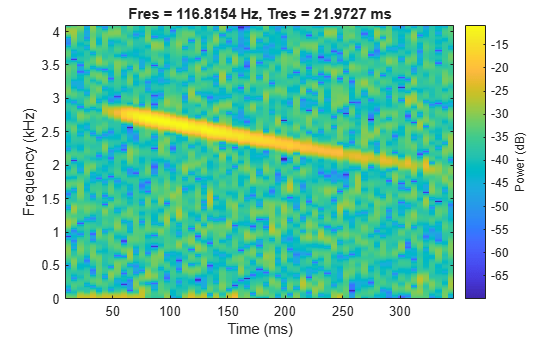`fsst(yChirp,Fs,'yaxis')`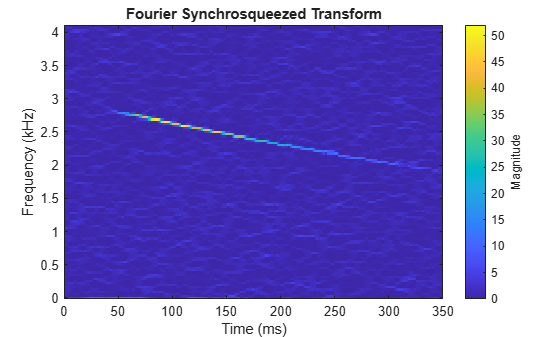```[sst,f] = fsst(yChirp,Fs); [fridge, iridge] = tfridge(sst,f,10); helperPlotRidge(yChirp,Fs,fridge);```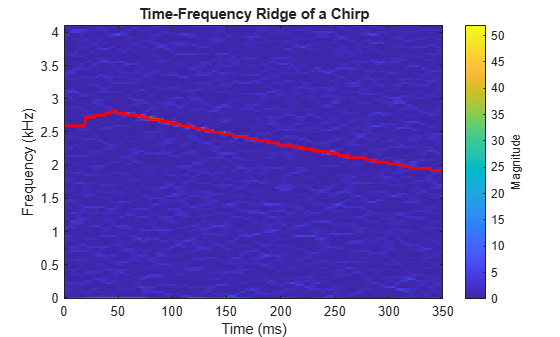```yrec = ifsst(sst,kaiser(256,10),iridge,'NumFrequencyBins',1); pspectrum(yrec,Fs,'spectrogram','MinThreshold',-70)```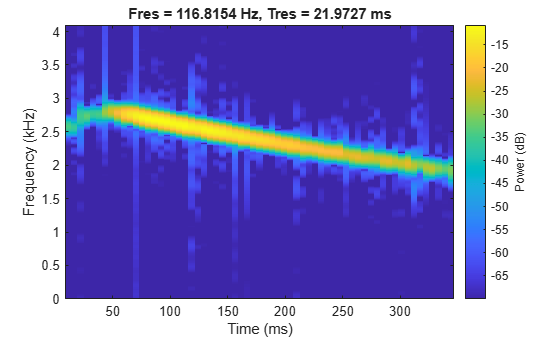```p = audioplayer([yChirp;zeros(size(yChirp));yrec],Fs,16); play(p);```

### 测量功率

```Fs = 1e8; bw = 60e6; t = 0:1/Fs:10e-6; IComp = chirp(t,-bw/2,t(end), bw/2,'linear',90)+0.15*randn(size(t)); QComp = chirp(t,-bw/2,t(end), bw/2,'linear',0) +0.15*randn(size(t)); IQData = IComp + 1i*QComp; segmentLength = 128; pspectrum(IQData,Fs,'spectrogram','TimeResolution',1.27e-6,'OverlapPercent',90)```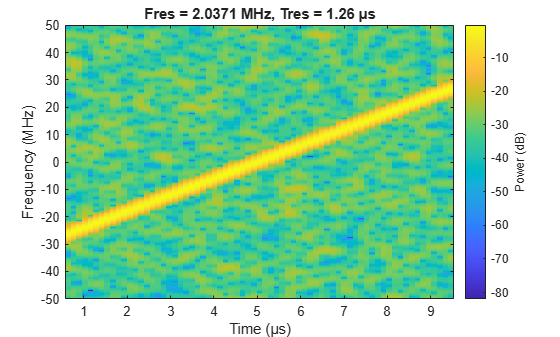### 对数频率尺度可视化

```Fs = 1e3; t = 0:1/Fs:10; fo = 10; f1 = 400; y = chirp(t,fo,10,f1,'logarithmic'); pspectrum(y,Fs,'spectrogram','FrequencyResolution',1, ... 'OverlapPercent',90,'Leakage',0.85,'FrequencyLimits',[1 Fs/2])```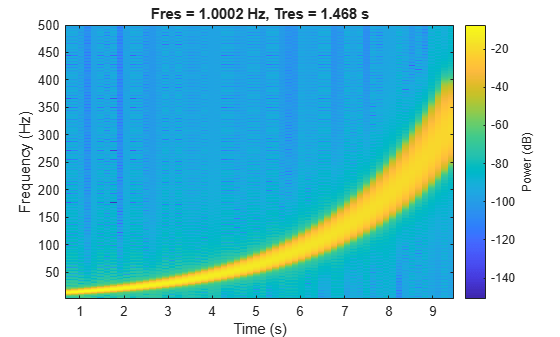```ax = gca; ax.YScale = 'log';```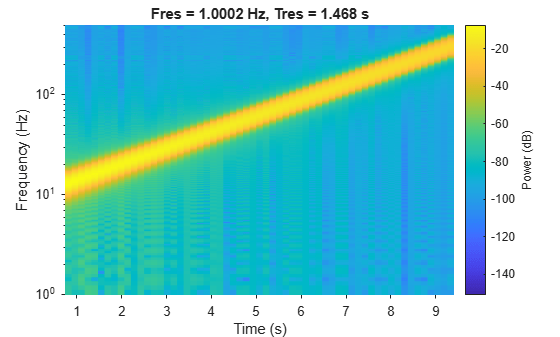### 三维瀑布可视化

```Fs = 10e3; t = 0:1/Fs:2; x1 = vco(sawtooth(2*pi*t,0.5),[0.1 0.4]*Fs,Fs); pspectrum(x1,Fs,'spectrogram','Leakage',0.8)```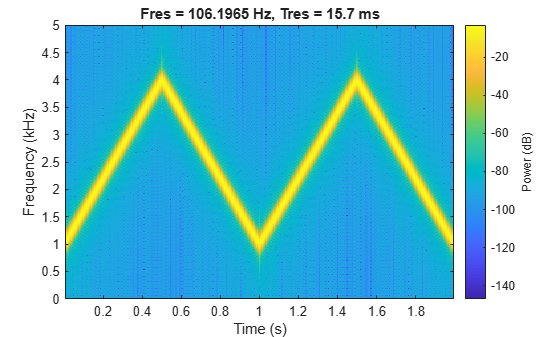```view(-45,65) colormap bone```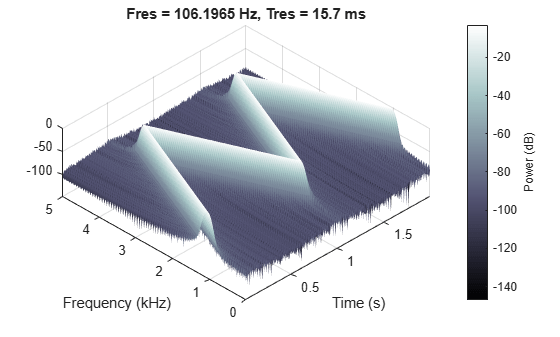### 使用持久频谱查找干扰

```fs = 1000; t = (0:1/fs:500)'; x = chirp(t,180,t(end),220) + 0.15*randn(size(t));```

```idx = floor(length(x)/6); x(1:idx) = x(1:idx) + 0.05*cos(2*pi*t(1:idx)*210);```

`pspectrum(x,fs,'FrequencyLimits',[100 290])`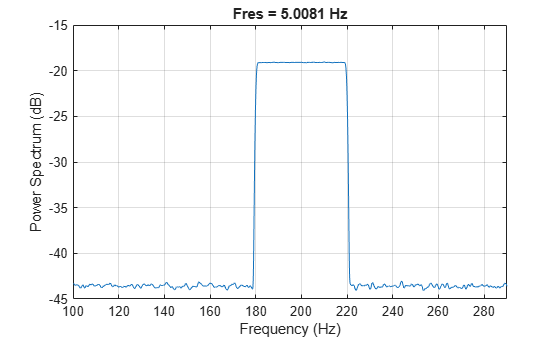```figure colormap parula pspectrum(x,fs,'persistence','FrequencyLimits',[100 290],'TimeResolution',1)```